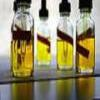#### You may also like### Mathematical Issues for Chemists

A brief outline of the mathematical issues faced by chemistry students.### Reaction Rates

Explore the possibilities for reaction rates versus concentrations with this non-linear differential equation### Blood Buffers

Investigate the mathematics behind blood buffers and derive the form of a titration curve.

# Catalyse That!

### Why do this problem?

This task is an exercise in understanding rates of change, graphs and (possibly) calculus in a way which does not use the usual route of speed-time graphs.

### Possible approach

The question will need to be read carefully, as it involves the rates of change as used in chemistry. Can learners explain what is happening clearly? Having a good mental image of the process will aid in the solution of this problem. Getting a sense of the 'limits' of the problem will help: what happens with a very, very fast rate of heating? What happens with a very, very slow rate of heating? By understanding these processes, it should become clear that there will be a rate which will minimise the time taken for the reaction to produce 100 mol.

### Key questions

The key questions should be used to help learners to understand that the amount catalysed equals the area under the graph of Rate against Time. For the straight line graphs involved here, simple calculation of areas will be enough. Learners should be given plenty of thinking or discussion time before being prompted with these key questions:
• What does the graph of rate of reaction against temperature look like?
• Will the reaction always lead to at least 100 mol being catalysed?
• Why would a very fast rate of heating give rise to a very small amount of chemical being catalysed?
• If the oven heated the compound to exactly 21 degrees, how long would it take to catalyse 100 moles?
• If the oven heated the compound at 1 degree per minute, how much would be catalysed in total?
• If the oven heated the compound at 2 degrees per minute, how much would be catalysed in total?

### Possible extension

The extension in the question suggests using non-constant rates of heating. There are several possible levels of engagement with this: How can you phrase the question in this case? How would the charts change qualitatively (draw a sketch)? Can you write down the equations? Can you solve them to find the answer?

### Possible support

First draw charts of temperature against time and rate against temperature for a fixed rate of 1 degree per minute. From these we need to work out a graph of rate against time. Try to get learners to solve this part first and then repeat for a 2 degree per minute rate.